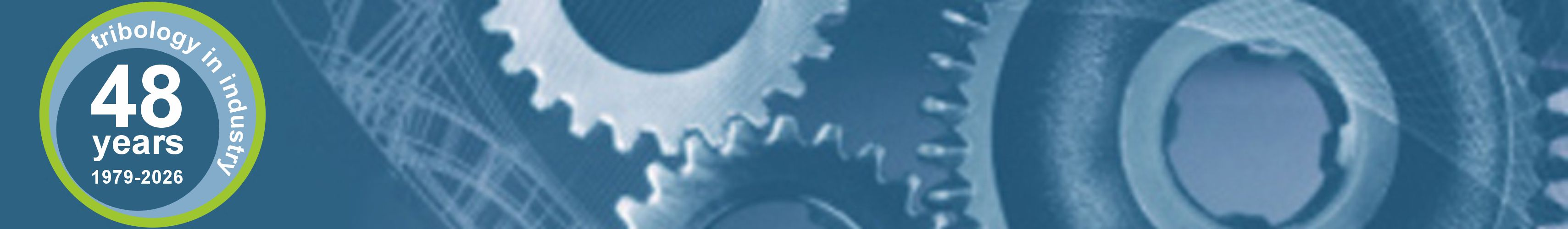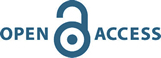# Volume 40, No 4, 2018, Pages 565-573

Comparative Analysis of Multiple Linear Regression and Artificial Neural Network for Predicting Friction and Wear of Automotive Brake Pads Produced from Palm Kernel Shell

Authors:

K.K. Ikpambese*, E.A. Lawrence

Abstract:

In this study, comparative analysis of multiple linear regression (MLR) and artificial neural network (ANN) for prediction of wear rate and coefficient of friction brake pad produced from palm kernel shell was carried out. The inputs parameters used for the two models generated using inertia dynamometer were the percentages of palm kernel shell, aluminium oxide, graphite, calcium carbonate, epoxy resin, interface temperature of the brake pad, and work done by brake application. Two model equations were developed using MLR model for predicting wear rate and coefficient of friction while the neural network architecture BR 7 [5-3] 2 was used to predict wear rate and coefficient of friction. The predicted wear rate and coefficient of friction by MLR model were compared with ANN model along with the measured values using statistical tools such as means square absolute error (MAE), root means square error (RMSE), and Nash-Scutcliffe efficiency (NSE). The results revealed that the MLR model outsmarts the ANN model with the values of MAE and RMSE reasonably low and NSE reasonably higher. The best MAE and RMSE values of 0.000 were observed at the three values of measured wear rates and coefficient of friction that matched with the predicted values using MLR compared to -0.0300 and 0.0740 for ANN model. However, the ANN model was equally found suitable for the prediction of wear rate and coefficient of friction of brake pads developed. The implication of these results is that the two models have the capabilities of being used simultaneously when estimating the wear and coefficient of friction of brake pads.

Keywords:

Multiple linear regression, Artificial neural network, Wear rate, Coefficient of friction, Inertia dynamometer

# Last EditionVolume 42
Number 4
December 2020# Announcements

TiI news RSS 2.0Table of contents